High School Math : Geometry

Example Questions

What is the area of a circle with a radius of?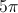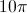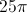Explanation:

To find the area of a circle you must plug the radius into the following equation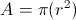In this case the radius isso we plug it in and square it to get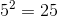We then multiply it byto get our answer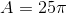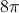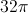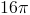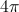Explanation:

Each set of two circles fits perfectly between the sides of the square. The diameters of two of the circle must equal the length of one side of the square.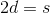Each side has a length of 8 and the diameter of a circle is twice the radius. We can use these relationships to substitute into the first equation.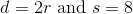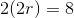Solve for the radius,.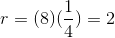The area of a circle can be calculated using the equation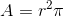. The shaded region is made of four circles, all with the same radius.

The area of one circle is given below.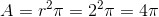The area of the shaded region (four circles) would be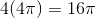.

O, M, and I represent three circles. Each circle shares the same center point. What is the area of the shaded region?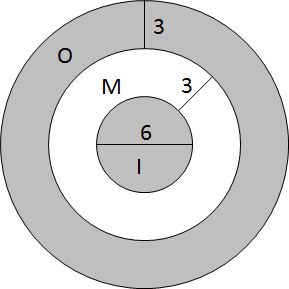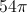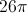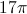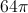Explanation:

The area of the shaded region can be found by using the areas of the individual circles. The area of the full figure is the area of circle O. The area of the center shaded area is the area of circle I. Circle M contains circle I. The area of the shaded region will be equal to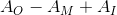.

First, find the area of each circle using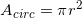.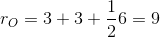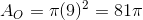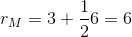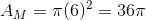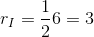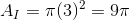Now we can substitute into our equation.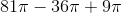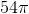What is the area of a circle with a diameter of?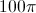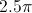Explanation:

The formula for area of a circle is. Unfortunately, the problem gives us a diameter instead of a radius. The good news is that the diameter is equal to twice the length of the radius or, mathematically,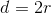Plug in the given diameter to find the radius: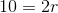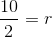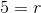Plug that into our first equation to solve: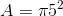Example Question #61 : Geometry

This figure is a circle with a radius of 3 cm.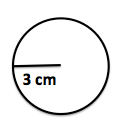What is the area of the circle (cm2)?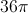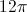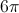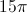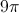Explanation:

The area of the circle istimes radius squared (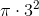).

Example Question #6 : How To Find The Area Of A Circle

If a circle has circumference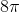, what is its area?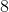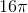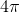Explanation:

If the circumference is, then since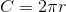we know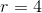. We further know that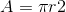, so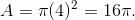Example Question #7 : How To Find The Area Of A Circle

If the equation of a circle is (x – 7)+ (y + 1)= 81, what is the area of the circle?

6561π

81π

18π

49π

81π

Explanation:

The equation is already in a circle equation, and the right side of the equation stands for r2 → r= 81 and r = 9

The area of a circle is πr2, so the area of this circle is 81π.

Example Question #8 : How To Find The Area Of A Circle

Assume π = 3.14

A man would like to put a circular whirlpool in his backyard. He would like the whirlpool to be six feet wide. His backyard is 8 feet long by 7 feet wide. By state regulation, in order to put a whirlpool in a backyard space, the space must be 1.5 times bigger than the pool. Can the man legally install the whirlpool?

No, because the area of the backyard is smaller than the area of the whirlpool.

Yes, because the area of the whirlpool is 28.26 square feet and 1.5 times its area would be less than the area of the backyard.

No, because the area of the backyard is 30 square feet and therefore the whirlpool is too big to meet the legal requirement.

No, because the area of the whirlpool is 42.39 square feet and 1.5 times its area would be greater than the area of the backyard.

Yes, because the area of the whirlpool is 18.84 square feet and 1.5 times its area would be less than the area of the backyard.

Yes, because the area of the whirlpool is 28.26 square feet and 1.5 times its area would be less than the area of the backyard.

Explanation:

If you answered that the whirlpool’s area is 18.84 feet and therefore fits, you are incorrect because 18.84 is the circumference of the whirlpool, not the area.

If you answered that the area of the whirlpool is 56.52 feet, you multiplied the area of the whirlpool by 1.5 and assumed that that was the correct area, not the legal limit.

If you answered that the area of the backyard was smaller than the area of the whirlpool, you did not calculate area correctly.

And if you thought the area of the backyard was 30 feet, you found the perimeter of the backyard, not the area.

The correct answer is that the area of the whirlpool is 28.26 feet and, when multiplied by 1.5 = 42.39, which is smaller than the area of the backyard, which is 56 square feet.

Example Question #9 : How To Find The Area Of A Circle

There are two identical circles on a plane that overlap. The radius of both circles is 1. The region in which they overlap has an area of π.

What is the total area of the 2 overlapping circles?

2π

0

π

2

1

π

Explanation:

The total area of both circles is π + π = 2π

Since the region overlaps, we cannot count it twice, so we must subtract it.

we get 2π – π = π

Example Question #10 : How To Find The Area Of A Circle

A square with a side length of 4 inches is inscribed in a circle, as shown below. What is the area of the unshaded region inside of the circle, in square inches?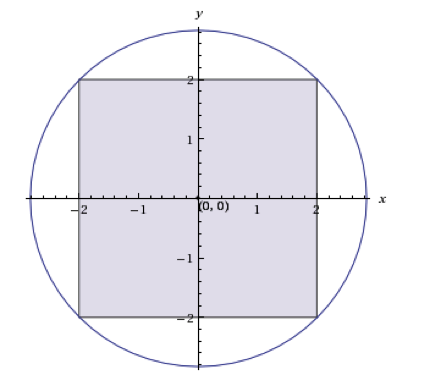4π-4

8π-4

8π - 16

8π-8

2π-4